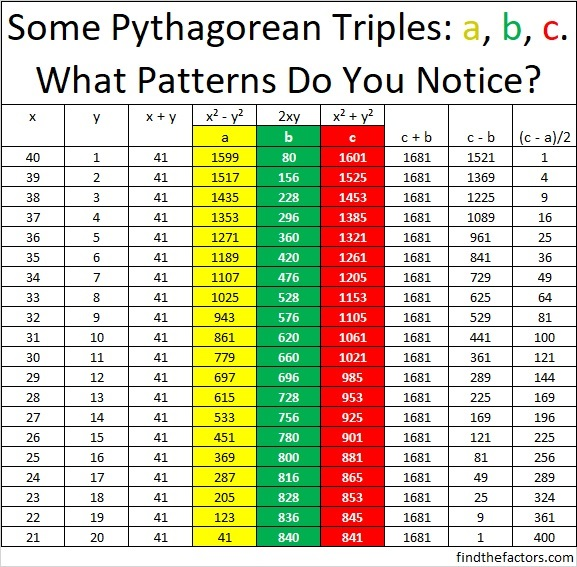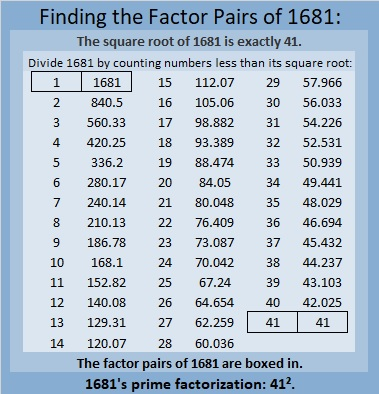# What Is 1681’s Claim to Fame?

Here are some more examples:

The sum of the even leg and hypotenuse for all ppts (primitive Pythagorean triples) is the square of an odd number. This is a rule for ppts that has been sadly overlooked. Because of this, you can find several ppts that have the sum of the even leg and hypotenuse with the same numerical value. Example (35,12,37), (21,20,29), (7,24,25). If their sum is the square of an odd number, p, the number of ppts is (p-1)/2 ppts. If the sum of the square of an odd number is not prime, then (p-1)/2 Pythagorean triples are produced.
Some are ppts and the others are scalar multiples. Example 15 will produce 4 ppt and 3 scalar multiples.

Since for ppts, the sum of the even leg and the hypotenuse is an odd number squared, and an odd number is the sum of an even and an odd number. (m+n)squared gives the m, n parts for the hypotenuse and even leg of ppts.

Wow, thanks! So since 15² = 225, the seven Pythagorean triples whose even side added to the hypotenuse equals 225 are (197-28-197), (165-52-173), (135-72-153), (105-88-137), (75-100-125), (45-108-117), and (15-112-113). I just had to find them myself after finding this out!

Glad you were able to get the 7 triples. The rule for odd numbers is an odd number is the sum of an even and an odd. I did show that the even side 2mn and hypotenuse m²+ n² is m²+n²+2mn which is (m+n)², m+n is odd.

M+N =15 is an equation with two variables. However, each has to be an integer. Thus (m,n) are (14,1) (13,2) (12,3) (11,4) (10,5) (9,6) (8,7). Note 3 sets of(m,n) have gcfs and these will be the non ppts.

The last pair of (8,7) has m=n+1. In your output (15, 112,113) you will note that 15 is now your odd leg., and the difference between the hypotenuse and the even leg is also 1.

This leads to a very interesting rule, which is

If the difference between the hypotenuse and even leg of a ppt is1. Then the area divided by the perimeter of the ppt is (odd leg-1)/4. Since your odd leg is 15, the ratio is(15-1)/4= 3.5

Now if the odd leg is a prime number then only one ppt is formed eg( 7,24,25).
All composite or multiples will produce more than one Pythagorean triple, one of which will produce a ppt with the hypotenuse minus the even leg equal 1,
For example 9 will be ( 9, 40,41) with m= n+1 .The other (9,12,15).
We can simply square 9 so (81+1)/2 =41 and(81-1)/2=40 are the hypotenuse and even leg respectively.

Or To get the the m and n ,m =(x+1)/2, n=(x-1)/2 . Thus x= 9 gives m=5 and n=4

So for the odd legs starting with the series of odd numbers 3,5,7,9…….. and
Applying the above rule where the difference between the hypotenuse and even leg is1

The area/ perimeter = (x-1)/4 we will get an arithmetic series with first term 0.5 and a common difference of 0.5

Using the formula, 3 gives 0.5, 5(1),7(1,5), 9 (2) ………
Thus producing the arithmetic series.

To develop the formula, Let x be the length of the odd leg. The hypotenuse plus the even leg is x² with the hypotenuse (x²-1)/2 and the even leg (x²-1)/2. Thus the perimeter is x +x² or x(x+1)
The area is x (x²-1)/4 or x(x+1)(x-1)/4

Area divided by perimeter (x-1)/4. This formula can be used by kids as young as grade 7.

The next formula is area/perimeter = n/2. Where is equal to m minus one

You can develop this formula starting with the hypotenuse m²+n² minus the even leg 2mn which leads to (m-n)²=1 thus (m-n)=1

Since the odd leg is m²-n² which is (m+n)(m-n) =(m+n)

I will leave the rest to any interested person.

Thank you for explaining more. This will be my topic when I write my 1681st post in a couple of months. (I should have said 7 months!) I will share your comments in that post so hopefully, more people will see them. Thanks again!

If the odd leg of a ppt is a prime number where its rightmost digit is 1 or 9 then the even leg is a multiple of 60. As you showed (11, 60, 61). (19, 180, 181). Some of the hypotenuses will also be a prime number. Of course, the even leg is a multiple of 4 (2mn) and either m or n is an even number.

The uniqueness of the A²+B² = C² formula is that it holds for any A and B and C. The Pythagorean triples are a special case where all three are integers. The amazing truth is for all As, Bs and Cs the three sides of a right triangle where A is greater than B. (a+b)² +(a-b)² = 2(c)²

Example 3²+4²=5² , (3+4)² + (4-3)²= 2(5)²

Algebraically (a+b)²+(a-b)² is 2a²+2b² which is 2c² and thus diving by 2 gives the original formula.

Can exponents greater than 2 produce a similar result.?

Let us assume a³+ b³= c³ a>b. And a and b are integers

Then (a+b)³+(a-b)³ is 2a³ + 6ab². Is 6ab² = 2b³?

Thus 6ab²-2b³=0 and 2b²(3a-b)=0. Either b=0 or b = 3a. If b=0 then a³=c³. If b=3a then a³+(3a)³=28a³=c³
The cube root of 28 is not an integer and thus c is not an integer.

Let us consider a⁴+b⁴=c⁴ then

(a+b)⁴ +(a-b)⁴ = 2a⁴+12a²b²+2b⁴. The extra term must be equal to zero to have the equation equal to 2c⁴. Thus either a =0 or b=0. Thus the extra term shows that c cannot be an integer in the original fourth-degree equation.

All higher degrees behave like n equal 3 for odd exponents and n equal 4 for even exponents.

Did Fermat have a simple proof? He did not have the tools available to Prof. Wiles.

That was wonderfully proven!

### A Puzzle with Pythagorean Triples:

Whether or not you were able to follow all that Duncan Fraser wrote, fill in the blanks on this next puzzle. Seriously, you should be able to complete it in less than a couple of minutes!Now sit back, relax, take notice and wonder about the patterns in this table:Mathematics is truly a thing of beauty!

### Factors of 1681:

• 1681 is a composite number and is a perfect square.
• Prime factorization: 1681 = 41 × 41 which can be written 1681 = 41².
• 1681 has at least one exponent greater than 1 in its prime factorization so √1681 can be simplified. Taking the factor pair from the factor pair table below with the largest square number factor, we get √1681 = (√41)(√41) =
• The exponent in the prime factorization is 2. Adding one to that exponent, we get (2 + 1) = 3. Therefore 1681 has exactly 3 factors.
• The factors of 1681 are outlined with their factor pair partners in the graphic below.### More About the Number 1681:

1681 is the sum of two squares:
40² + 9² = 1681.

1681 is the hypotenuse of two Pythagorean triples:
369-1640-1681, which is 41 times (9-40-41).
720-1519-1681, calculated from 2(40)(9), 40² – 9², 40² + 9².

1680, 1681, 1682, 1683, and 1684 are the second smallest set of FIVE consecutive numbers whose square roots can be simplified.### Puzzle Solution:All of the numbers are perfect squares AND concatenations of exactly two perfect squares:
7² = 49; 2² = 4, 3² = 9.
13² = 169; 4² = 16, 3² = 9.
19² = 361; 6² = 36, 1² = 1.
35² = 1225; 1² = 1, 15² = 225.
38² = 1444; 12² = 144, 2² = 4.
41² = 1681; 4² = 16, 9² = 81.
57² = 3249; 18² = 324, 3² = 9.
65² = 4225; 2² = 4, 15² = 225.
70² = 4900; 2² = 4, 30² = 900.

1681 shares that claim to fame with those other numbers, but is the only one on the list that is a concatenation of two 2-digit squares.

This site uses Akismet to reduce spam. Learn how your comment data is processed.